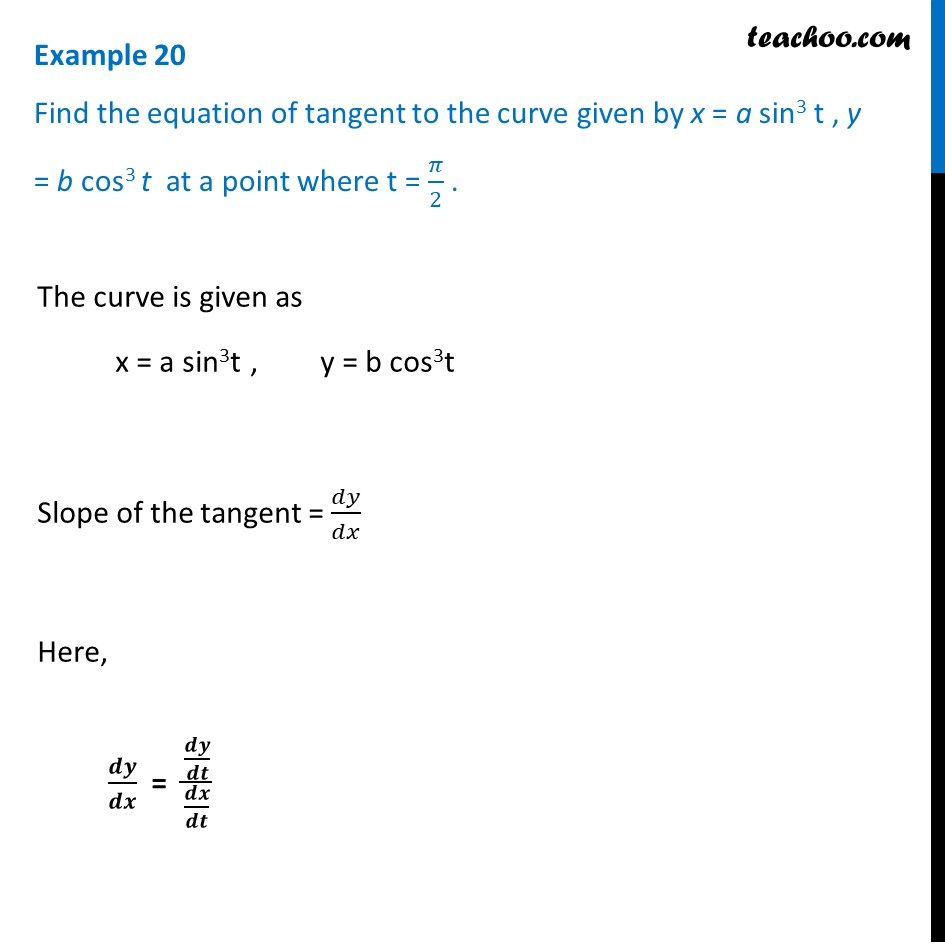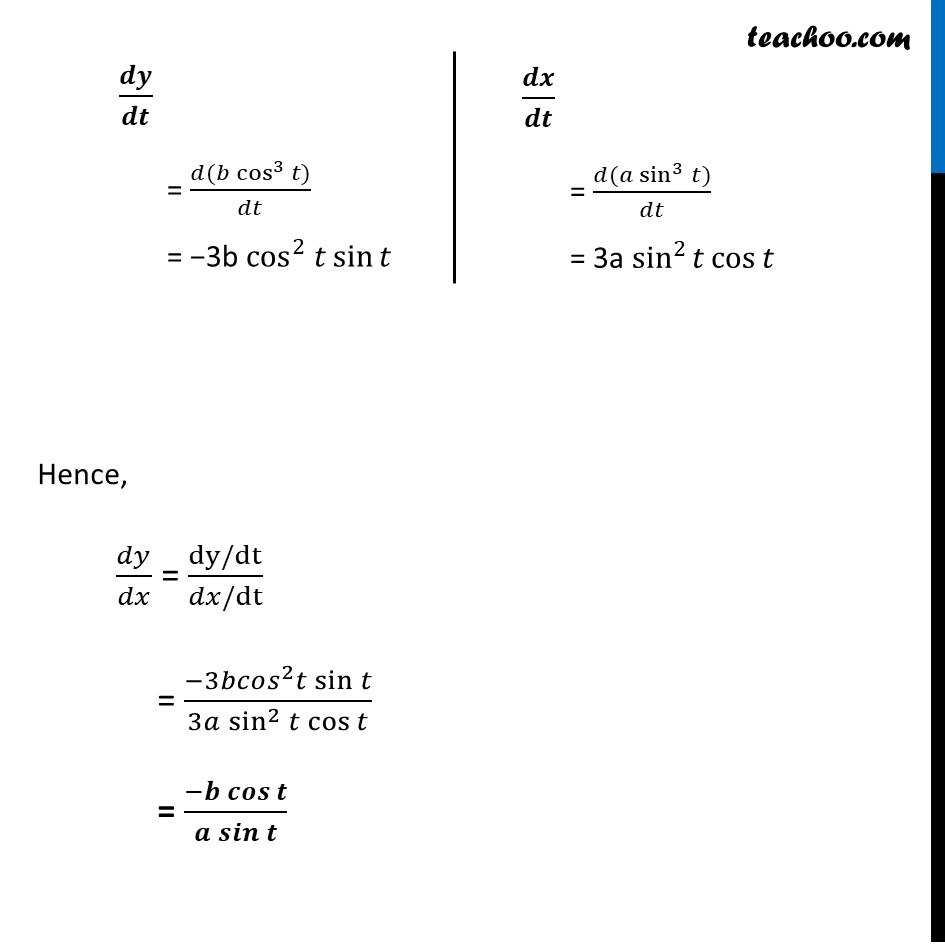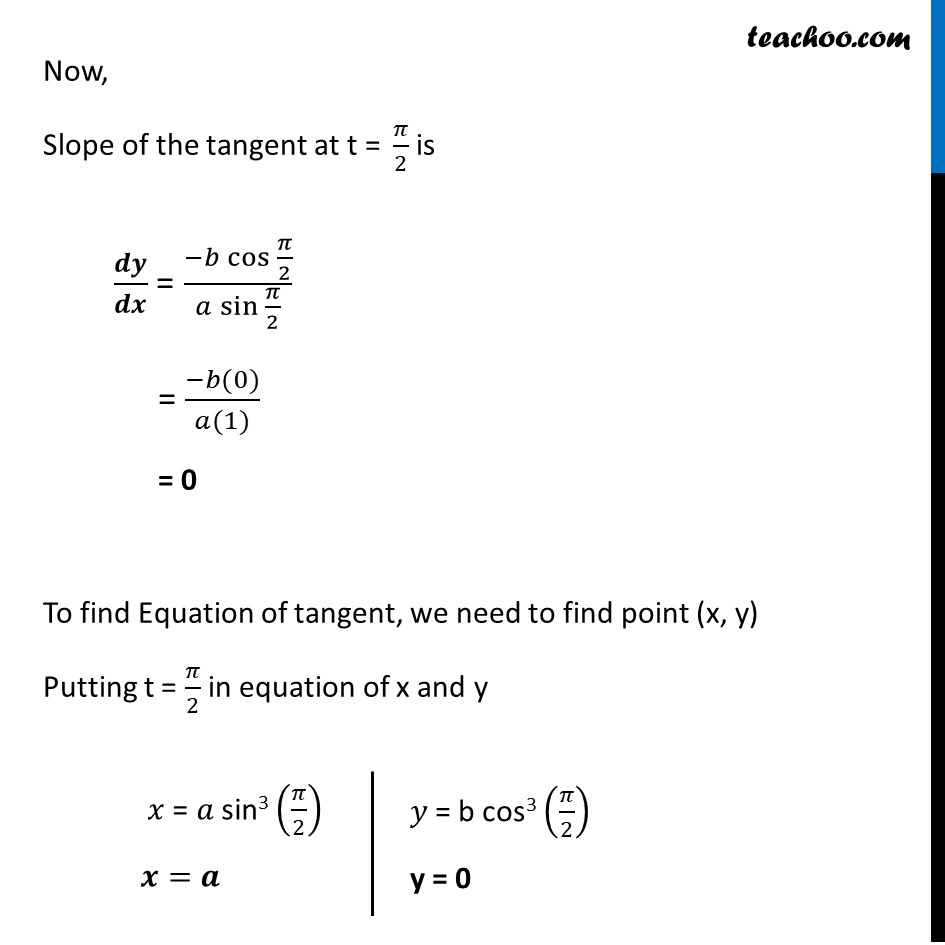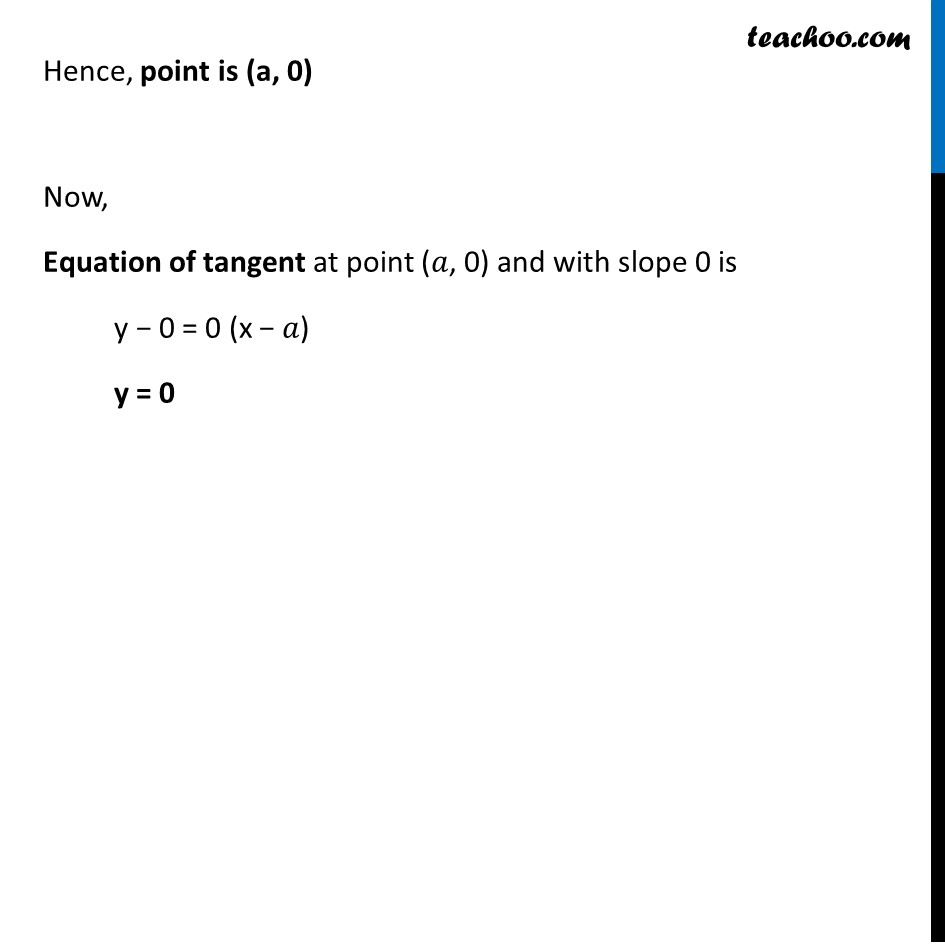Finding equation of tangent/normal when point and curve is given

Chapter 6 Class 12 Application of Derivatives
Concept wiseLearn in your speed, with individual attention - Teachoo Maths 1-on-1 Class

### Transcript

Question 7 Find the equation of tangent to the curve given by x = a sin3 t , y = b cos3 t at a point where t = 𝜋/2 . The curve is given as x = a sin3t , y = b cos3t Slope of the tangent = 𝑑𝑦/𝑑𝑥 Here, 𝒅𝒚/𝒅𝒙 = (𝒅𝒚/𝒅𝒕)/(𝒅𝒙/𝒅𝒕) 𝒅𝒚/𝒅𝒕 = (𝑑(𝑏 cos^3⁡〖𝑡)〗)/𝑑𝑡 = −3b cos^2 𝑡 sin⁡𝑡 𝒅𝒙/𝒅𝒕 = (𝑑(𝑎 sin^3⁡〖𝑡)〗)/𝑑𝑡 = 3a sin^2⁡𝑡 cos⁡𝑡 Hence, 𝑑𝑦/𝑑𝑥 = (dy/dt)/(𝑑𝑥/dt) = (−3𝑏𝑐𝑜𝑠^2 𝑡 sin⁡𝑡)/(3𝑎 sin^2⁡〖𝑡 cos⁡𝑡 〗 ) = (−𝒃 𝒄𝒐𝒔⁡𝒕)/(𝒂 𝒔𝒊𝒏⁡𝒕 ) Now, Slope of the tangent at "t = " 𝜋/2 is 𝒅𝒚/𝒅𝒙 = (−𝑏 〖cos 〗⁡〖𝜋/2〗)/(𝑎 〖sin 〗⁡〖𝜋/2〗 ) = (−𝑏(0))/(𝑎(1)) = 0 To find Equation of tangent, we need to find point (x, y) Putting t = 𝜋/2 in equation of x and y 𝑥 = 𝑎 sin3 (𝜋/2) 𝒙=𝒂 𝑦 = b cos3 (𝜋/2) y = 0 Hence, point is (a, 0) Now, Equation of tangent at point (𝑎, 0) and with slope 0 is y − 0 = 0 (x − 𝑎) y = 0